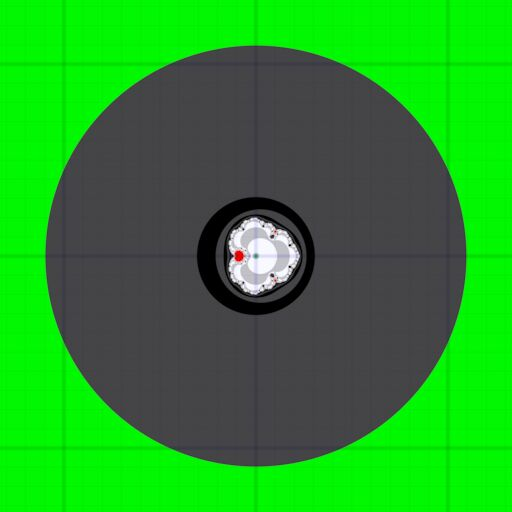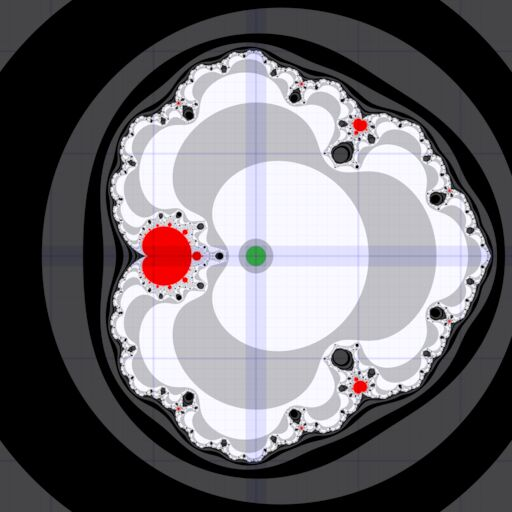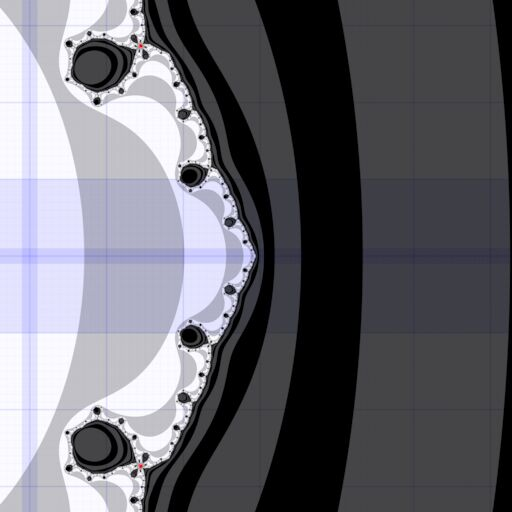# # z→z²+cz⁵

mick asked a math.stackexchange.com question about the filled-in Julia sets of the polynomial:

$f_c(z) = z^2 + c z^5$

## # 1 Critical Points

$f_c'(z) = 2 z + 5 c z^4 = 0$

has 4 solutions

$z_0 = 0 \\ z_0 = \sqrt{-\frac{2}{5c}}$

which is where iteration should start from to determine the number of components that the filled-in Julia set has (1 if all critical points remain bounded, infinitely many if a critical point escapes to infinity).

$$z_0 = 0$$ implies $$z_n = 0$$ for all $$n \ge 0$$, which is not interesting.

## # 2 Boundedness

Suppose $$|z_n| < r$$. If that implies $$|z_{n+1}| < r$$, then $$|z_m| < r$$ for all $$n > m$$ (by induction).

We want $|z_{n+1}| = |z_{n}^2 + c z_{n}^5| < |z_{n}| + |c| |z_n|^5 < r^2 + |c| r^5 < r$ whence $|c| < \frac{1 - r}{r^4}$

Suppose $|z_0| = \sqrt{\frac{2}{5|c|}} < r$ whence $\frac{2}{5r^3} < |c|$

Maximizing $$r$$ such that $\frac{2}{5r^3} < |c| < \frac{1 - r}{r^4}$ gives $r < \frac{5}{7},$ that is, if $$|z_{n}| < \frac{5}{7}$$ for any $$n$$ then it’ll remain bounded.

Additionally, if $|c| > \frac{2}{5\left(\frac{5}{7}\right)^3} = \frac{686}{625} = 1.0976$ all the critical points are certainly bounded, because $$|z_0| < \frac{5}{7}$$.

Note: this does not imply it converges to $$0$$, but the technique of the next section could maybe be adapted. I think boundedness is enough but I’m not 100% sure.

## # 3 Escape To Infinity

Assume $$|c| \le \frac{686}{625}$$.

Suppose $$|z_n| > (1 + \epsilon) R$$ with some $$\epsilon > 0$$. If that implies $$|z_{n+1}| > (1 + \epsilon)^2 R$$, then $$|z_{n + m}| > (1 + \epsilon)^{m+1} R \to \infty$$ as $$m \to \infty$$.

Concretely, let $$R = \frac{1}{\sqrt{|c|}}$$ and $$\epsilon = \frac{4}{15}$$.

Writing $$|c| = \frac{1}{b^k}$$ for some $$k$$ and $$b > 1$$, then $$R = b^{k/3}$$. Now

\begin{aligned} |z_{n+1}| &= |z_n^2 + c z_n^5| \\ &> \left| |z_n|^2 - |c| |z_n|^4 \right| \\ &> \left| (1 + \epsilon)^2 R^2 - |c| (1 + \epsilon)^5 R^5 \right| \\ &= (1 + \epsilon)^2 \left| b^{2k/3} - (1+\epsilon)^3 \frac{b^{5k/3}}{b^k} \right| \\ &= (1 + \epsilon)^2 ((1 + \epsilon)^3 - 1) b^{2k/3} \\ &= (1 + \epsilon)^2 R \times ((1 + \epsilon)^3 - 1) R \end{aligned}

In the second part of the product, substitute $$\epsilon = \frac{4}{15}$$, and having assumed $$|c| \le \frac{686}{625}$$ we know $$R \ge \sqrt{\frac{625}{686}}$$, that is,

\begin{aligned} |z_{n+1}| &> (1 + \epsilon)^2 R \times \frac{3484}{3375} \sqrt{\frac{625}{686}} \\ &= (1 + \epsilon)^2 R \times 1.000744019\ldots \\ &> (1 + \epsilon)^2 R \end{aligned}

Therefore, if $$|c| \le \frac{686}{625} = 1.0976$$ then $|z_n| > \frac{19}{15 \sqrt{|c|}}$ implies that that the iteration escapes to infinity.

Moreover, if $$0 < |c| < 0.01$$, then $$|z_1| > \frac{19}{15 \sqrt{|c|}}$$, that is this region of the $$c$$ plane always escapes to infinity (for $$z_0$$ a non-zero critical point). The bound 0.01 could be increased with more effort.

## # 4 Special c

The right-most tip of the escaping region (see visualisation below) is pre-periodic, with positive real $$c$$ satisfying $$f_c(f_c(z_0(c))) = f_c(z_0(c))$$ for the real critical point and $$f_c(f_c(f_c(z_0(c)))) = f_c(z_0(c))$$ for the complex critical points (which means all the critical points are bounded and the filled-in Julia set has a single component).

Using SageMath, the exact value can be found as the cube of a root of a quintic with algebraic coefficients:

f(c, z) = z^2 + c * z^5
for cp in solve(diff(f(c, z), z) == 0, z):
try:
g(c) = (f(c, f(c, z)) - f(c, z)).substitute(cp).expand()
P = sum([ QQbar(H) * polygen(QQbar)^H for H in h(x).coefficients() ])
c0 = QQbar.polynomial_root(P, CIF(RIF(0.6, 0.7), RIF(-0.1, 0.1)))^3
z0 = QQbar(- (2 / (5 * c0))^(1/3))
z1 = QQbar((- 2 / (5 * c0))^(1/3))
print(all([f(c0, z0) == f(c0, f(c0, z0)), f(c0, z1) == f(c0, f(c0, f(c0, z1)))]))
print(h(x))
print(P)
print(CIF(c0))
except:
pass


True
-3/1953125*5^(2/3)*(15625*5^(2/3)*2^(2/3)*x^5 - 18750*2^(1/3)*x^3 - 648*2^(1/3))
-0.3257301139913888?*x^5 + 0.1061001071608432?*x^3 + 0.003666819703478739?
0.2294487977025897?


that is, the special $$c$$ is $$c = 0.229448797702589\ldots$$.

I used SageMath version 9.5, Release Date: 2022-01-30 as found in Debian Bookworm (current testing distribution) on 2023-03-09.

## # 5 Visualisation

Source code for Fragmentarium (FragM fork version Fragmentarium 2.5.7.221026 Digilantism with include path Examples/Claude): z2cz5.frag

• green: critical point proven to escape to infinity (center) or remain bounded (outside)
• red: no decision reached after 1000 iterations
• light: critical point escapes to infinity (filled-in Julia set has infinitely-many components)
• dark: critical point remains bounded (filled-in Julia set has a single component)
• blue coordinate grid at 1, 0.1, 0.01, … spacing

Wide view:Zoomed view:Close up view of special $$c$$:Animation of filled-in Julia set near special $$c$$ for real $$c \pm \frac{1}{10}$$: